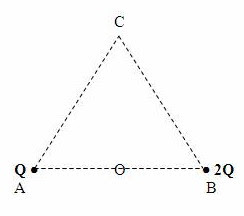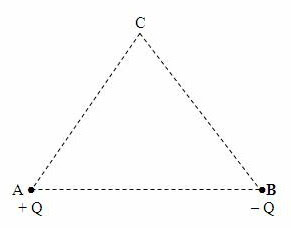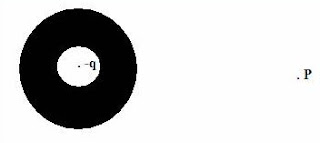## Saturday, March 03, 2012

### Electrostatics - Multiple Choice Questions on Electric Field and Potential

Today we will discuss a few simple, but interesting, multiple choice questions on electrostatics. Here are the questions:(1) ABC is an equilateral triangle. When positive charges Q and 2Q are placed at points A and B, the electric potential at the mid point (O) of AB is found to be 180 V. What is the electric potential at the vertex C of the triangle under this condition?

(a) 60 V

(b) 90 V

(c) 120 V

(d) 180 V

(e) 240 V

The distance of the vertex C of the triangle from the charges is twice the distance from the point O. Since the electric potential due to a point charge is inversely proportional to the distance, the potential at the vertex C must be half the value at C. So the answer is 90 V.

[Mathematically, you will write (with usual notations)

(1/4πε0)(3Q/r) = 180 and

(1/4πε0)(3Q/2r) = x

This gives x = 90 V](2) Equal and opposite point charges + Q and Q are fixed at the corners A and B of an equilateral triangle (Fig.). If a negative point charge (– q) is placed at the vertex C of the triangle, the net force on this charge is directed

(a) towards right

(b) towards left

(c) towards A

(d) towards B

(e) towards the mid point of side AB

The force on the charge q due to the charge + Q is attractive and is directed along CA. The force due to the charge – Q is repulsive and is directed along BC. Since these forces have the same magnitude, their resultant acts parallel to BA. The net force on the charge – q is thus directed leftwards [Option (b)].

(3) A cube of side 4 cm has a constant electric potential of 2 volt on its surface. If there are no charges inside the cube, the potential at a distance of 1 cm from the centre of the cube is

(a) zero

(b) 0.25 V

(c) 0.5 V

(d) 1 V

(e) 2 V

Since the surface of the cube is an equipotential surface and there are no charges inside the cube, the electric field inside the cube must be zero. This means that the electric potential everywhere inside the cube is 2 V itself [Option (e)].(4) A spherical conductor of radius R has a central cavity (Fig.) with a negative point charge (q) located at the centre of the cavity (without touching the surface of the cavity). The electric fiel at a point P distant r from the centre of the sphere is

(a) q/4πε0r2 directed towards the sphere

(b) q/4πε0r2 directed away from the sphere

(c) q/4πε0r2 directed upwards

(d) q/4πε0r2 directed downwards

(e) zero

The negative charge at the centre of the cavity will induce positive charge q on the surface of the cavity and negative charge –q on the outer surface of the sphere. The situation is similar to that of a spherical conductor carrying a charge –q so that the magnitude E of the electric field at the point P is given by

E = q/4πε0r2

Since the field is due to negative charge, the direction of the field is towards the centre of the sphere.